# 14.2.3 Ratio, Rates and Proportions II, PT3 Focus Practice

Question 10:
Diagram below shows the distance from Town P to Town Q and Town Q to Town R.(a) Rahim rode his bicycle from Town P at 9.00 a.m. and took 2 hours to reach Town Q.
What is the speed, in km/h, of the bicycle?
(b) Rahim took 30 minutes rest at Town Q and continued his journey to Town R three times faster than his earlier speed.
State the time he reached Town R.

Solution:
(a)

(b)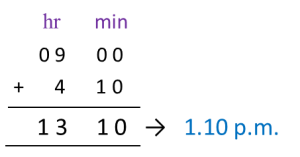Question 11:
Diagram below shows a trailer travelling from a factory to location P and location P to location Q. The trailer departs at 8.00 a.m.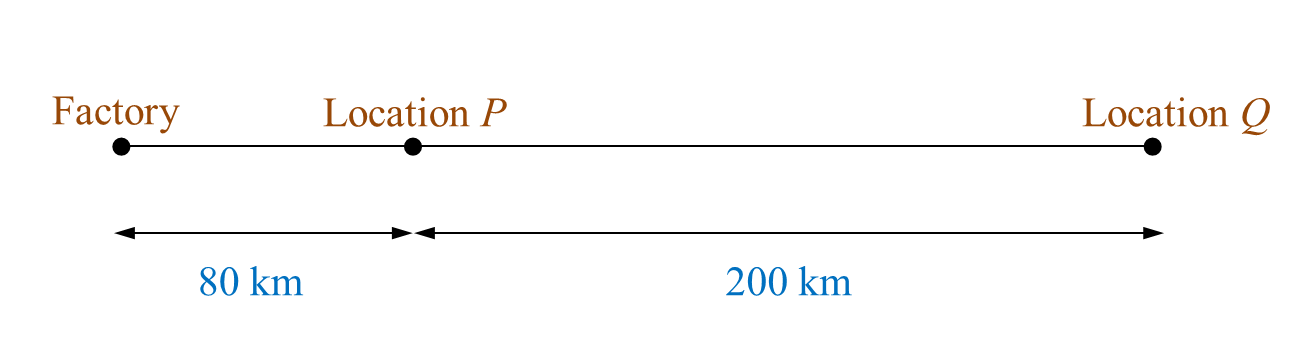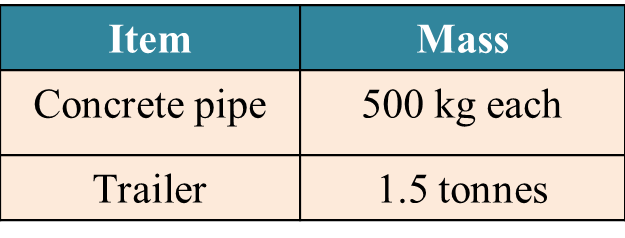(a) Based on the Table, calculate the total mass, in tonne, of the trailer and its load.
(b) The trailer arrived at location P at 10.00 a.m. and it stopped for 1½ hours to unload half of the concrete pipes. The trailer then continued its journey to location Q two times faster than its earlier speed. State the time, the trailer reached at location Q.

Solution:
(a)

(b)Question 12:
Diagram below shows travel information of Jason and Mary from Town A to Town B. Jason drives a lorry while Mary drives a car.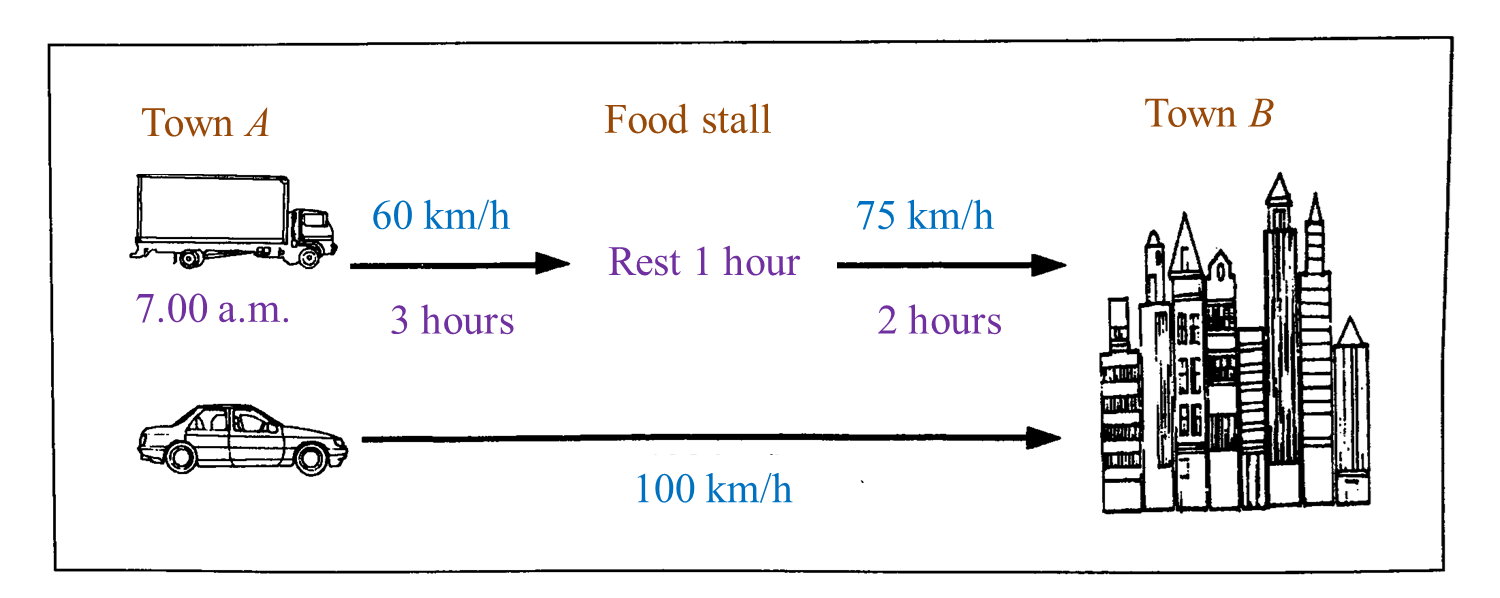(a) Jason started his journey from Town A at 7.00 a.m.
State the time, Jason reached at Town B.
(b) If both of them reached Town B at the same time, state the time Mary started her journey from Town A.

Solution:
(a)
Total time taken from Town A to Town B
= 3 hours + 1 hour + 2 hours
= 6 hours

Time Jason reached Town B
= 0700 + 0600
= 1300 hours → 1.00 p.m.

(b)# 14.2.2 Ratio, Rates and Proportions II, PT3 Focus Practice

Question 6:
Mei Ling drives her car from Kuantan to Kuala Terengganu for a distance of 170 km for 2 hours. She then continues her journey to Kota Bahru and increases her speed to 100 kmh-1 for 45 minutes.
Calculate the acceleration, in kmh-2, of the car.

Solution:

Question 7:
Diagram shows the distance between K and L.A car moves from K to L with an average speed of 80 kmh-1. After rest for 1 hour 30 minutes, the car then returns to K. The average speed of the car from L to K increases 20%. If the car reaches K at 5 p.m., calculate the time the car starts its journey from K.

SolutionThe car starts its journey from K at 11:50 a.m.

Question 8:
Mr Wong is going to watch a movie at 2.30 p.m at a cinema that is 60 km away from his house. He leaves at 1.25 p.m and drives at an average speed of 70 km/h for half an hour. If he drives at an average speed of 75 km/h for the remaining journey, will he arrive before the movie starts?

Solution

Question 9:
Karen drives her car from town P to town Q at an average speed of 80 km/h for 2 hours 15 minutes. She continues her journey for a distance of 90 km from town Q to town R and takes 45 minutes.
Calculate the average speed, in km/h, for the journey from P to R.

Solution:

# 14.2.1 Ratio, Rates and Proportions II, PT3 Focus Practice

Question 1:
The distance from town A to town B is 120 km. A car leaves town A for town B at 11.00 a.m. The average speed is 80 km h-1 .
At what time does the car arrive at town B.

Solution:

Question 2:
Kenny drives his car from town P to town Q at a distance of 180 km in 3 hours.
Faisal takes 30 minutes less than Kenny for the same journey.
Calculate the average speed, in km/h, of Faisal’s car.

Solution:

Question 3:
Rafidah drives her car from town L to town M at an average speed of 90 km/h for 2 hours 40 minutes. She continues her journey for a distance of 100 km from town M to town N and takes 1 hour 20 minutes.
Calculate the average speed, in km/h, for the journey from L to M.

Solution:

Question 4:
Susan drives at an average speed of 105 km/h from town F to town G.
The journey takes 3 hours.
Susan takes 30 minutes longer for her return journey from town G to town F. Calculate the average speed, in km/h, for the return journey.

Solution:

Question 5:
Table below shows the distances travelled and the average speeds for four vehicles.

 Vehicle Distance (km) Average speed (km/h) A 230 115 B 250 100 C 170 85 D 245 70
Which vehicles took the same time to complete the journey?

Solution:

Thus, the vehicles A and C took the same time to complete the journey.

# 13.2.3 Graphs of Functions, PT3 Focus Practice

Question 7:
Use graph paper to answer this question.
Table below shows the values of two variables, x and y, of a function.The x-axis and the y-axis are provided on the graph paper on the answer space.
(a) By using a scale of 2 cm to 5 units, complete and label the y-axis.
(b) Based on the table above, plot the points on the graph paper.
(c) Hence, draw the graph of the function.Solution:Question 8:
Use graph paper to answer this question.
Table below shows the values of two variables, x and y, of a function.The x-axis and the y-axis are provided on the graph paper on the answer space.
(a) By using a scale of 2 cm to 2 units, complete and label the y-axis.
(b) Based on the table above, plot the points on the graph paper.
(c) Hence, draw the graph of the function.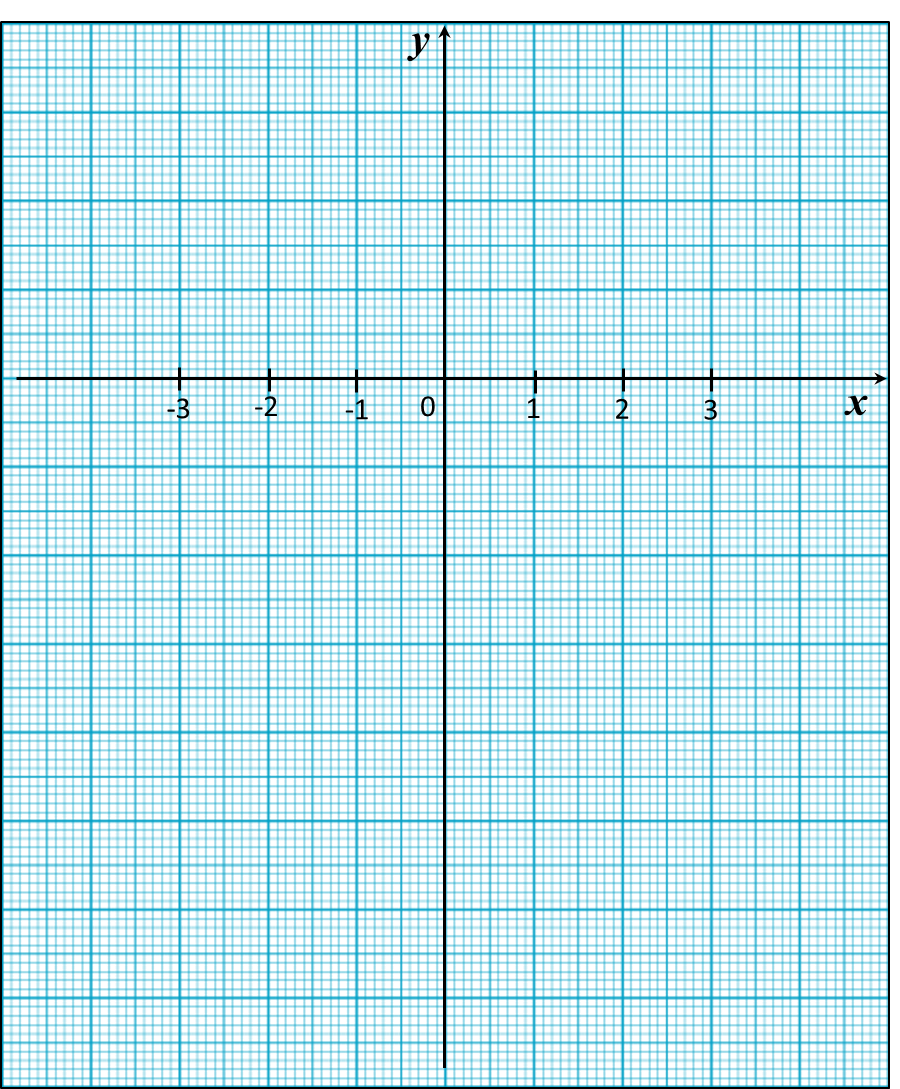Solution: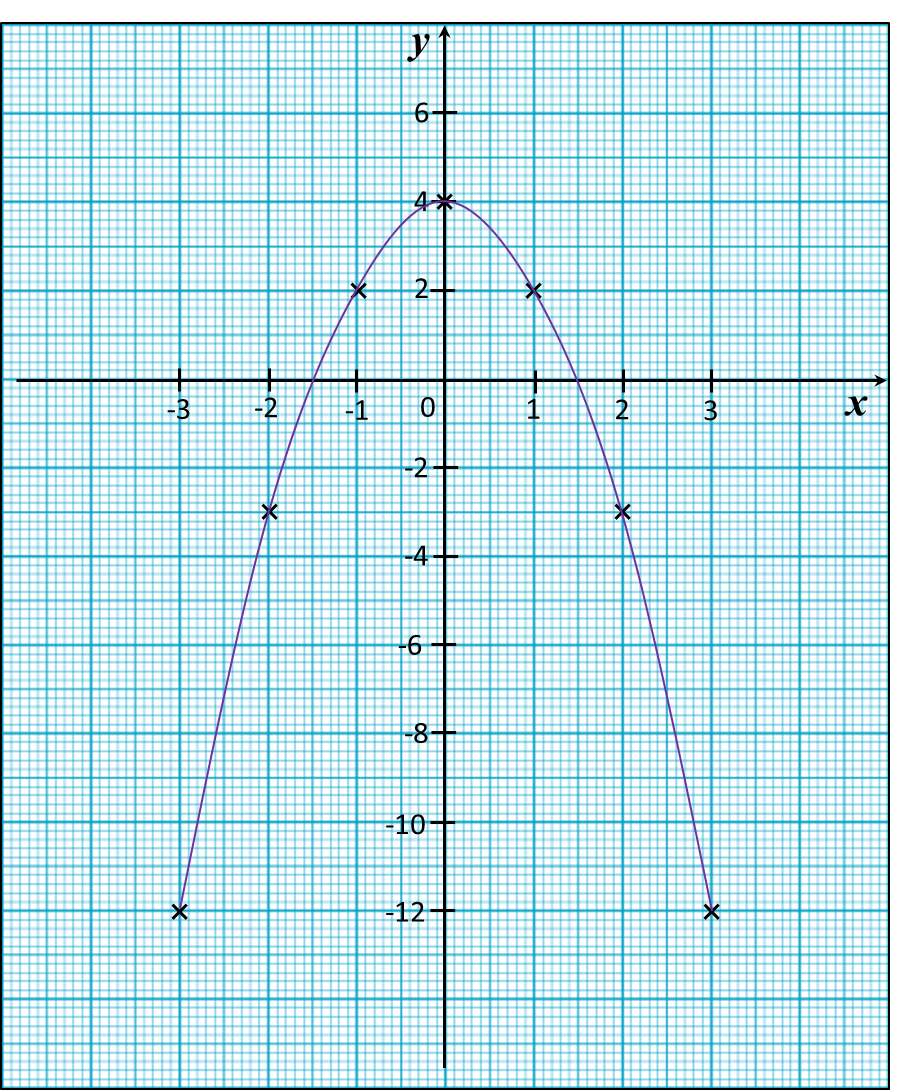# 13.2.2 Graphs of Functions, PT3 Focus Practice

 
 
13.2.2 Graphs of Functions, PT3 Focus Practice
 
 
Question 4:
 
Use graph paper to answer this question.
 
Table below shows the values of two variables, x and y, of a function.
 

 
 
   x     –3     –2     –1     0     1     2     3     y     –19     –3     1     –1     –3     1     17  
 
 
The x-axis and the y-axis are provided on the graph paper on the answer space.
 
(a)  By using a scale of 2 cm to 5 units, complete and label the y-axis.
 
(b) Based on the table above, plot the points on the graph paper.
 
(c)  Hence, draw the graph of the function.
 
 

 Answer: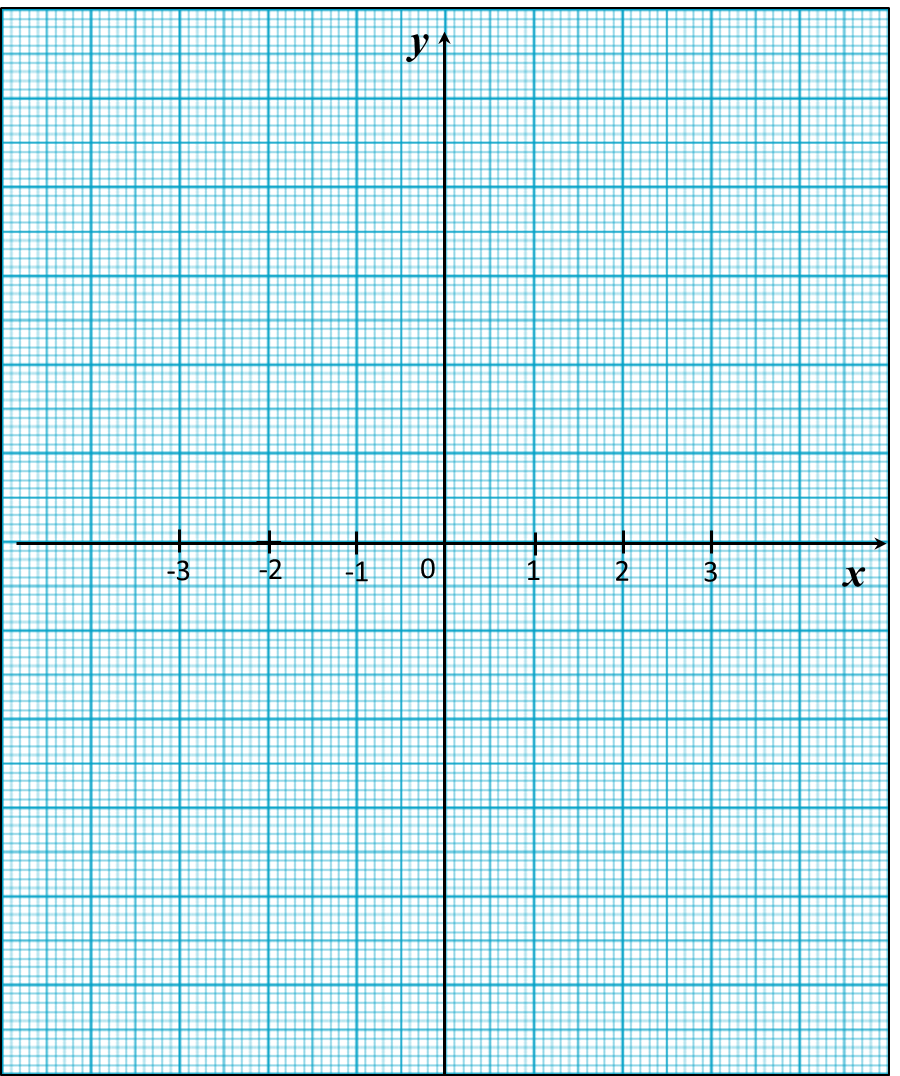Solution:Question 5:
 
Use graph paper to answer this question.
 
Table below shows the values of two variables, x and y, of a function.
 

 
 
   x     –4     –3     –2     –1     0     1     2     y     31     17     7     1     –1     1     7  
 
 
The x-axis and the y-axis are provided on the graph paper on the answer space.
 
(a)  By using a scale of 2 cm to 5 units, complete and label the y-axis.
 
(b)   Based on the table above, plot the points on the graph paper.
 
(c)  Hence, draw the graph of the function.Solution: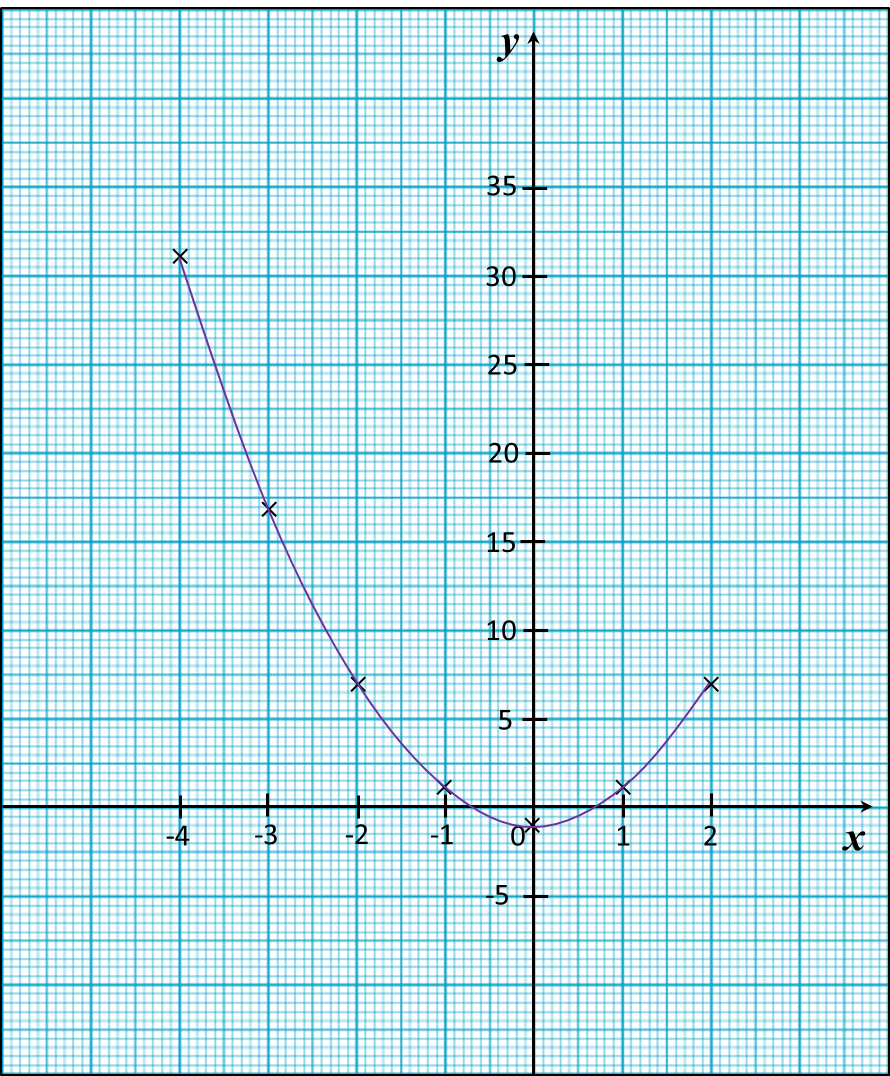Question 6:
(a) Complete table below in the answer space for the equation L = x2 + 5x by writing the value of L when x = 2.
(b) Use graph paper to answer this part of the question. You may use a flexible curve rule.
By using a scale of 2 cm to 1 unit on the x-axis and 2 cm to 5 units on the y-axis, draw the graph of L = x2 + 5x for 0 ≤ x ≤ 4.Solution:
(a)
When x = 2
L = 22 + 5(2)
= 4 + 10
= 14

(b)# 13.2.1 Graphs of Functions, PT3 Focus Practice

13.2.1 Graphs of Functions, PT3 Focus Practice

Question 1:

In the figure shown, when x = 4, the value of y is

Solution:

is the point on the graph where x = 4.
Hence, when x = 4, y = 5.

Question 2:
Diagram shows the graph of a function on a Cartesian plane.

The equation that represents the function is

Solution:
Use points (0, 6) and (-3, 0) to determine the function.

 Function Value of y when x = 0 x = –3 y = x + 3 3 0 y = x – 3 –3 –6 y = 2x + 6 6 0 (correct) y = 2x – 6 –6 –12

Question 3:
Table below shows the values of variables x and y of a function.

 x –2 0 1 y –6 2 3
Which of the following functions is satisfied by the ordered pair?
A = 7x + 2
B = x3 + 2
C = x + x2 – 2
D = x2 + 2

Solution:
Only y = x3 + 2 is satisfied by all the ordered pairs.
(i) –6 = (–2)3 + 2
(ii)   2 = 03 + 2
(iii)  3 = 13 + 2

# 13.1 Graphs of Functions

13.1 Graphs of Functions

13.1.1 Functions
1.   Function is a relationship that expresses a variable (dependent) in terms of another variable (independent).

2.   is a function of x if and only if every value of x corresponds to only one value of y.

13.1.2  Graphs of Functions
1.   A graph of function is a representation of the function as one or more lines on a coordinate plane.
Example:

2.
When drawing graphs of functions, it is best to observe these few points:
· choose suitable scales for both axes.
· use at least three points to draw a straight line to ensure accuracy.
· use at least five points to draw a curve to ensure accuracy.